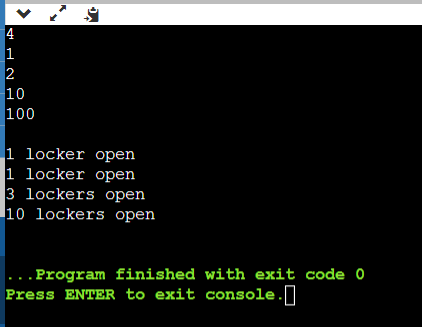# The box game in Java

## Question

A school has N students and N boxes, one box for every student. At a certain event, the teacher plays the following game: he asks the first student to go and open all the boxes. He then asks the second student to go and close all the even-numbered boxes. The third student is asked to check every third box. If it is open, the student closes it; if it is closed, the student opens it. The fourth student is asked to check every fourth box. If it is open, the student closes it; if it is closed, the student opens it. The remaining students continue this game. In general, the nth student checks every nth box. After all the students have taken their turn, some of the boxes are open and some are closed.

Your task is to write a program that reads the number of boxes in a school and outputs the number of boxes that are opened following the mechanics of the game.

Input: The first line takes in an integer M denoting the number of test cases. M number of lines follow. For each test case, the number of students is given.

Output: For each test case, you are to display “Case #X: “, followed by the number of opened boxes. In java

Sample Input

4
1
2
10
100

Sample Output

Case #1: 1 locker open
Case #2: 1 locker open
Case #3: 3 lockers open
Case #4: 10 lockers open

## Summary

In this question, there are N students and N boxes, and there is one technique or game rule given. And according to that game rule, the students should open and close the boxes. In the end, we have to tell that how many boxes are opened. In this question, we will take N as input and it will be the number of students and boxes also. And we have to write a java program for this game.

## Explanation

For calculating the number of boxes that are opened, the game rule is given as follows. Among the n students, 1st student opens all the boxes. 2nd student closes the even-numbered box, 3rd student opens every third box if it is close otherwise closes it if it is open. 4th student does the same thing but at every fourth box. And the remaining students follow this rule till the N-th student.

For a better understanding, if we take one example for 10 students. The following changes will be as follows at every step. For ten students there will be ten boxes.

 Round box-1 box-2 box-3 box-4 box-5 box-6 box-7 box-8 box-9 box-10 0 Close Close Close Close Close Close Close Close Close Close 1 Open Open Open Open Open Open Open Open Open Open 2 Open Close Open Close Open Close Open Close Open Close 3 Open Close Close Close Open Open Open Close Close Close 4 Open Close Close Open Open Open Open Open Close Close 5 Open Close Close Open Close Open Open Open Close Open 6 Open Close Close Open Close Close Open Open Close Open 7 Open Close Close Open Close Close Close Open Close Open 8 Open Close Close Open Close Close Close Close Close Open 9 Open Close Close Open Close Close Close Close Open Open 10 Open Close Close Open Close Close Close Close Open Close

In the 10th row, we can see there are only three boxes open.

## Code

The code for the box game in java is presented below.

import java.util.*;
class Main{
public static void main(String args[]){
Scanner a = new Scanner (System.in);

/* number of test cases tc*/
int tc;
tc=a.nextInt ();
int count []=new int [tc];

/* for evry test case*/
for(int m=0;m<tc; m++) {
/* number of students ns*/
int ns;
ns=a.nextInt ();
/* to have the ns number of lockers*/
int locker []=new int[ns];
/* to keep all the lockers closed initially */
for(int i=0;i<ns; i++)
locker[i]=0;
for (int i=0;i<ns; i++){
int k=i+1;
/* to alter every kth box, by kth student */
for(int j=k-1;j<ns; j+=k){
if(locker[j] ==1) locker [j]=0;
else locker[j] = 1;
}
}
/*to count number of open boxes*/
count [m]=0;
for(int i=0;i<ns;i++)
if(locker[i]==1)
count [m]++;
}
/* to display th output*/
System.out.println();
for(int i=0; i<tc;i++){
if (count [i]==1)
System.out.println(count[i]+" locker open");
else
System.out.println(count[i]+" lockers open");
}
}
}


## Output

The output for the box game in java is presented below.